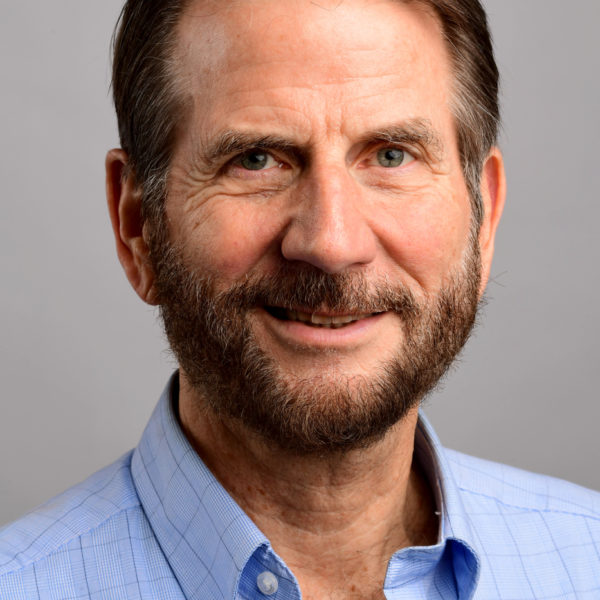# Robert McOwen

Professor

#### Expertise:

• Partial Differential Equations

Prof. McOwen studies partial differential equations, especially linear and nonlinear elliptic equations on noncompact domains and manifolds. He uses methods of functional analysis, especially weighted Sobolev spaces. Prof. McOwen has given applications to differential geometry, especially to the conformal scalar curvature equation, a semi-linear elliptic equation relating the scalar curvatures of two conformally-related Riemannian metrics, and also to the equations of fluid dynamics.

Received my Ph.D. from U.C. Berkeley in 1978, under the supervision of H.O. Cordes. After a one year NSF-AMS postdoctoral fellowship at NYU, I joined the Northeastern University Math Department in 1979.

Contact

567 LA (Lake Hall), Boston, MA 02115
Institutes, Labs & Research Centers
Differential Geometry Group
Mathematics

Differential geometry uses tools from analysis to study objects called manifolds that generalize Euclidean space.

Geometric Analysis Group
Mathematics

The Geometric Analysis Group falls under the field of mathematical analysis, which is a broad branch of mathematics that encompasses many fields, generally sharing a basis in calculus. Historically, analysis has played a crucial role in solving problems in physics and engineering; recent years have seen surprising applications to solve problems in other mathematical fields like the Poincaré Conjecture in topology.

Partial Differential Equations and Dynamical Systems Group
Mathematics

The Partial Differential Equations and Dynamical Systems Group falls under the field of mathematical analysis, which is a broad branch of mathematics that encompasses many fields, generally sharing a basis in calculus. Historically, analysis has played a crucial role in solving problems in physics and engineering; recent years have seen surprising applications to solve problems in other mathematical fields like the Poincaré Conjecture in topology.